# Python NetworkX – Tutte Graph

• Last Updated : 29 Apr, 2021

It is a graph with 46 vertices and 69 edges. It is important because it is an exception to Tait’s conjecture which states that every 3-regular polyhedron has a Hamiltonian cycle.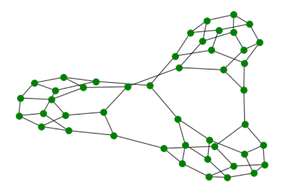Tutte Graph

Properties of Tutte Graph:

Attention geek! Strengthen your foundations with the Python Programming Foundation Course and learn the basics.

To begin with, your interview preparations Enhance your Data Structures concepts with the Python DS Course. And to begin with your Machine Learning Journey, join the Machine Learning - Basic Level Course

• It is a cubic polyhedral graph which is evident from the diagram above as it is both cubic and polyhedral
• It is a Non-Hamiltonian graph.
• It is a Planar Graph.
• The chromatic number of the Tutte graph is 3.
• It can be constructed by connecting the 3 Tutte fragments such that the resulting graph is s 3-connected and planar.
• A Diagram of the Tutte fragment is given below.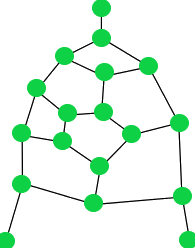Tutte Fragment

• It is evident from the above diagram that a Tutte fragment has 18 nodes.

We will use the networkx module for realizing a Tutte graph. It comes with an inbuilt function networkx.tutte_graph() and can be illustrated using the networkx.draw() method.

Syntax:

networkx.draw(G, node_size, node_color)

Parameters:

• G: It refers to the Tutte graph object
• node_size: It refers to the size of nodes.
• node_color: It refers to color of the nodes.

Below are some examples which depict how to illustrate a Tutte graph in Python:

Example 1:

## Python3

 `# import required module``import` `networkx` `# create object``G ``=` `networkx.tutte_graph()` `# illustrate graph``networkx.draw(G)`

Output: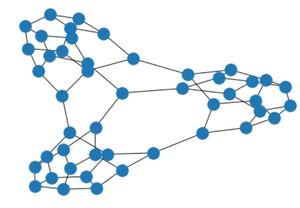Example 2:

## Python3

 `# import required module``import` `networkx` `# create object``G ``=` `networkx.tutte_graph()` `# illustrate graph``networkx.draw(G, node_color``=``'green'``)`

Output: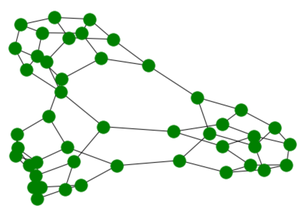Example 3:

## Python3

 `# import required module``import` `networkx` `# create object``G ``=` `networkx.tutte_graph()` `# illustrate graph``networkx.draw(G, node_size``=``15``,``              ``node_color``=``'green'``)`

Output: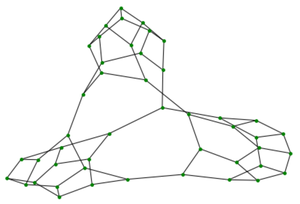Note: The shape of output graph illustration is generated randomly but the number, size and color of nodes will be according to the argument passed in networkx.draw() method.

My Personal Notes arrow_drop_up